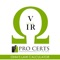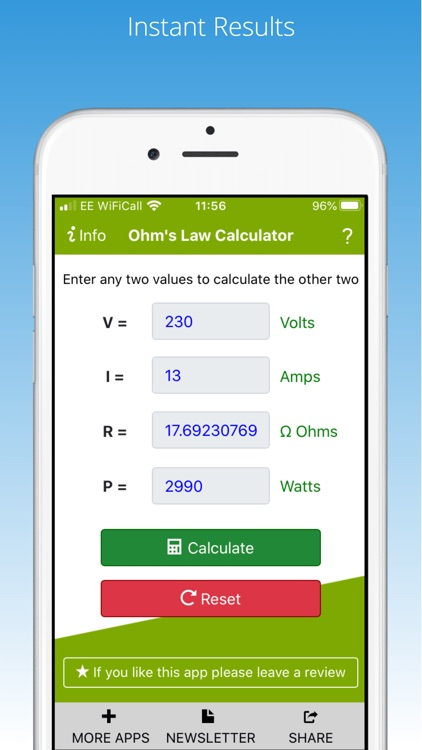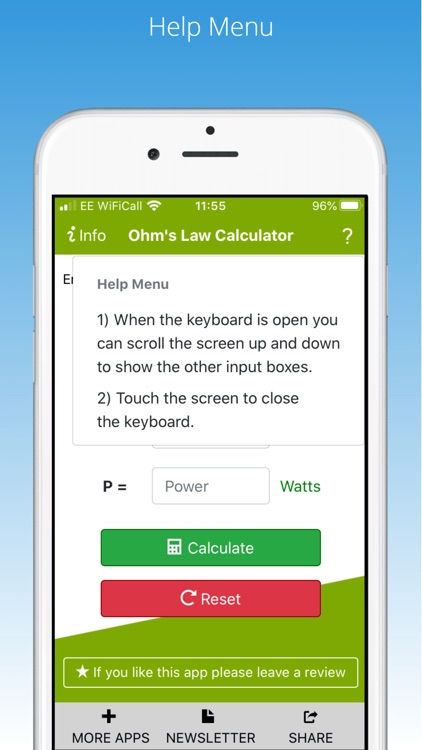## Ohms Law calculator for calculating V I R electrical formulas or Power, current, Resistance and Power# Ohms Law

by Pro Certs Software LtdOhms Law calculator for calculating V I R electrical formulas or Power, current, Resistance and Power.### App Details

Version
1.3.1
Rating
(1)
Size
11Mb
Genre
Utilities Productivity
Last updated
April 30, 2020
Release date
January 8, 2013

### App Screenshots### App Store Description

Ohms Law calculator for calculating V I R electrical formulas or Power, current, Resistance and Power.

Ohms Law Calculator Features:
- Calculate Voltage (volts)
- Calculate Current (amps)
- Calculate Resistance (ohms)
- Calculate Power (watts)

Input any two values to calculate the other two.

Ohms Law Formula:
V = Voltage
I = Current
R = Resistance
P = Power

An advanced Ohms Law calculator to calculate Voltage, Current, Resistance & Power.

How to use:
1) Enter any two values to calculate the other two.
2) Tap calculate.

Voltage Calculator | Current Calculator | Resistance Calculator | Power Calculator all in one app.

Ohms Law Calculator for iPhone and iPad.

Check out our collection of electrical apps for iPhone and iPad https://www.procertssoftware.com/apps

Disclaimer:
AppAdvice does not own this application and only provides images and links contained in the iTunes Search API, to help our users find the best apps to download. If you are the developer of this app and would like your information removed, please send a request to [email protected] and your information will be removed.